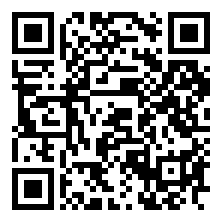kdwycz 8月 12, 2012
• 在其它设备中阅读本文章
•@李绵 学姐为了给我说明++ –和指针，给我了一道题

int a[]={1,2,3,4,5}. 最初p=a 依次写出 p (++p) (p)++ p p– –(p) p

1. p ：p指向a 所以p==1；
2. (++p)：p指向a 所以(++p)==2；
3. (p)++：p指向a并把a加一，因为++是后缀，所以此时(p)++==2； 之后a==3；
4. p ：p指向a 所以p==3；
5. p–：p指向a 以为–是后缀，所以 此时(p–) == a[1 ]== 3； 之后 p指向a；
6. –(p)：p指向a并把a减一，所以 –(p)==0；
7. p ：p指向a 所以p==0；